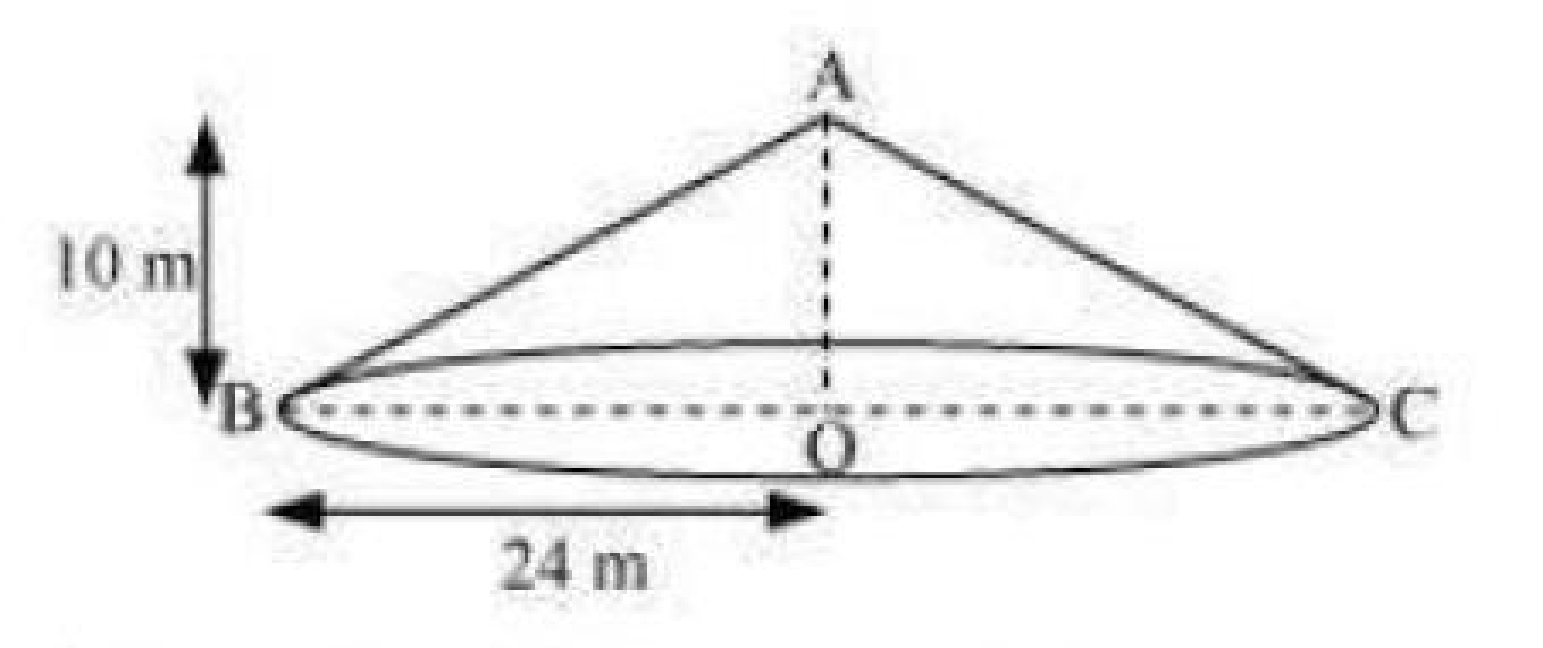Guru

# A conical tent is 10 m high and the radius of its base is 24 m. Find (i) slant height of the tent. (ii) cost of the canvas required to make the tent, if the cost of 1 m2 canvas is Rs 70. (Assume π=22/7) Q.4

• 0

Please suggest me the best way for solving the question of class 9th of surface areas and volumes chapter of math of question no. 4 of math, please give me the easiest way for solving the question of exercise 13.3 A conical tent is 10 m high and the radius of its base is 24 m. Find (i) slant height of the tent. (ii) cost of the canvas required to make the tent, if the cost of 1 m2 canvas is Rs 70. (Assume π=22/7)

Share

1.Let ABC be a conical tent

Height of conical tent, h = 10 m

Radius of conical tent, r = 24m

Let the slant height of the tent be l.

(i) In right triangle ABO, we have

AB2 = AO2+BO2(using Pythagoras theorem)

l2 = h2+r2

= (10)2+(24)2

= 676

l = 26

Therefore, the slant height of the tent is 26 m.

(ii) CSA of tent = πrl

= (22/7)×24×26 m2

Cost of 1 m2 canvas = Rs 70

Cost of (13728/7)m2 canvas is equal to Rs (13728/7)×70 = Rs 137280

Therefore, the cost of the canvas required to make such a tent is Rs 137280.

• 0